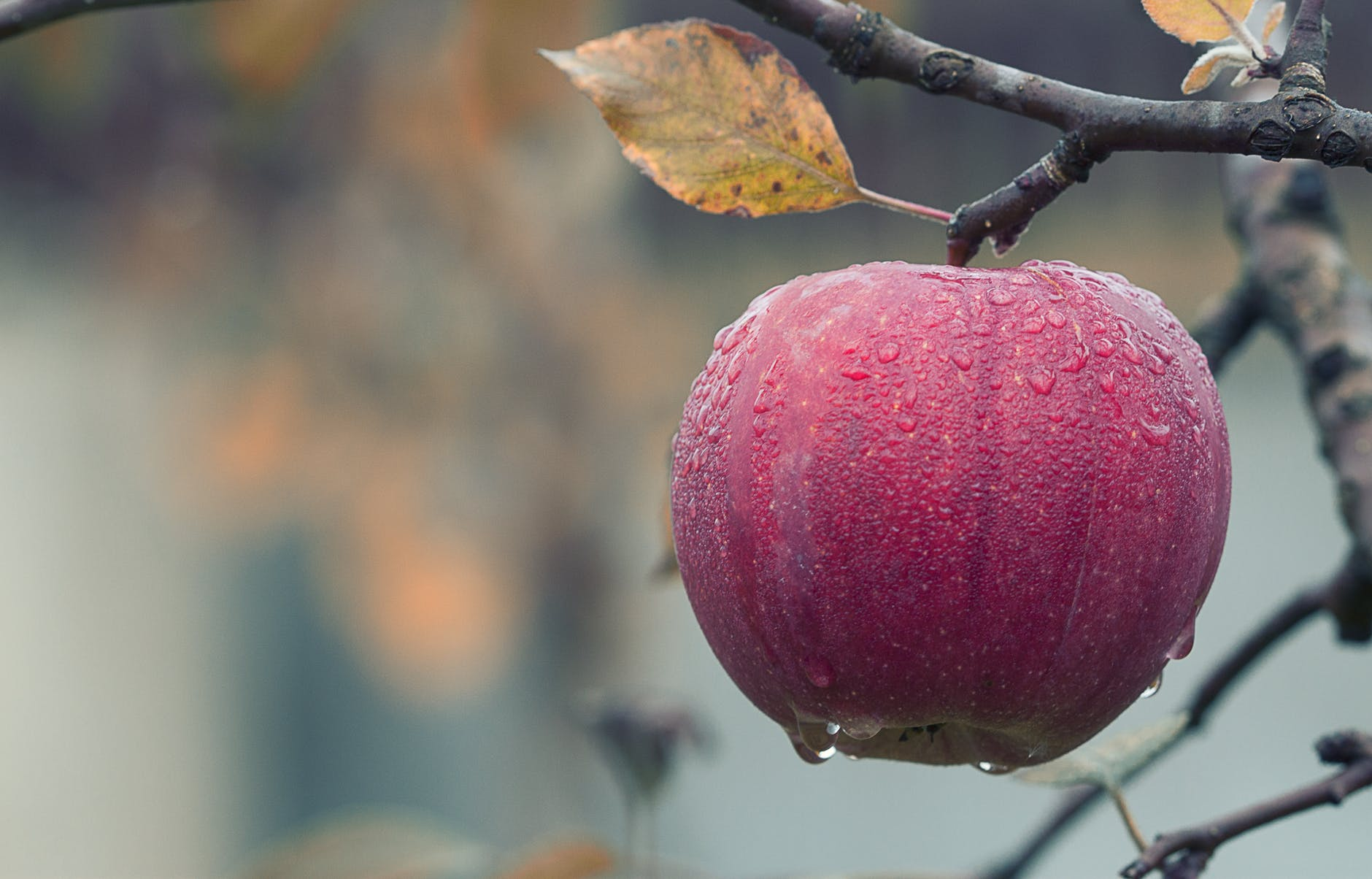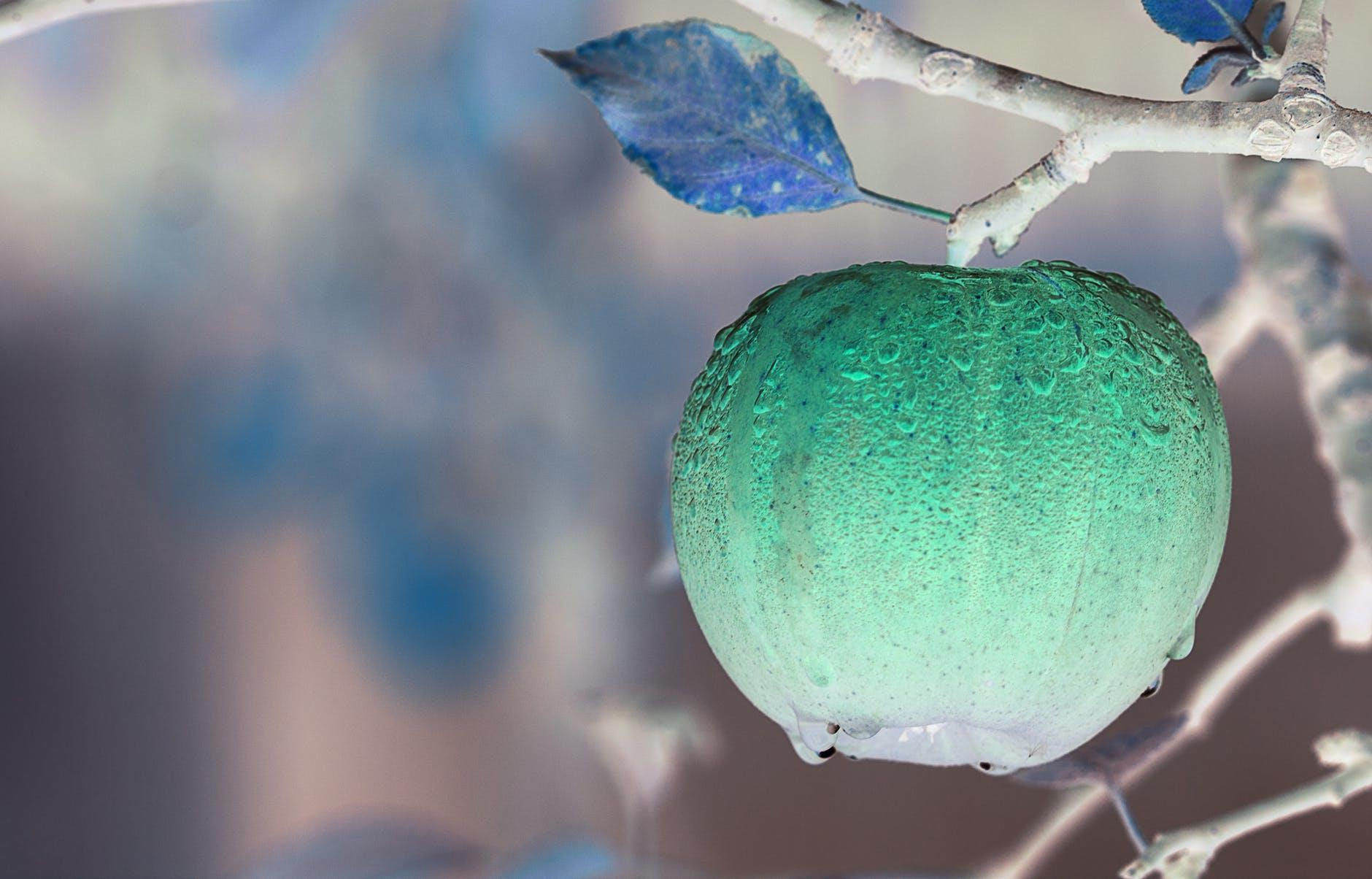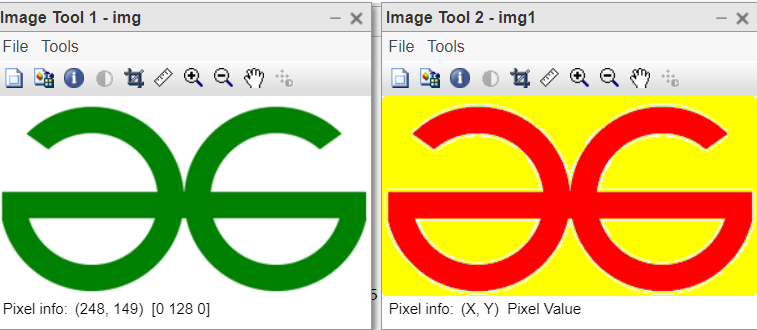# MATLAB | Complement colors in RGB Image

• Last Updated : 01 Jul, 2022

In MATLAB, an RGB image is basically a 3-D Image array ( M*N*3 ) of the color pixel, where each color pixel is associated with three values which correspond to red, blue, and green color components of RGB image at a specified spatial location. In complement of colors an RGB image, Each color in RGB image is replaced with their complementary color.

```For example,
red color ( 255, 0, 0) is replaced with cyan color ( 0, 255, 255 ).
blue color ( 0, 0, 255 ) is replaced with yellow color ( 255, 255, 0).
here, cyan is complementary color for red and yellow are complementary color for blue. ```

Dark areas become lighter and light areas become darker in RGB images as a result of complement.

Example:

## MATLAB

 `% MATLAB code read an RGB Image``img=imread(``'apple.jpg'``);` `% Complement colors of read RGB image``% Using imcomplement() function``comp=imcomplement(img);` `% Display Complemented Image``imshow(comp);`

Complementing colors of an RGB Image Without Using Library functions:

Complement an RGB image by subtracting each pixel value from the maximum pixel value supported by the class of RGB image and the difference is used as the pixel value in the complemented RGB image.

• If the RGB image belongs to class ‘uint8’, each pixel will have values between [ 0 – 255]. So, for the ‘uint8’ class type maximum value a pixel can have is 255. If an RGB image belongs to class ‘uint16’, each pixel will have values between [ 0 – 65535]. So, for the ‘uint16’ class type maximum value a pixel can have is 65535. Similarly, the maximum possible pixel value In the ‘double’ class type RGB image is 1.0.
For example, let an RGB image of ‘uint8’ class
• If an image pixel has a value of 127 then, in complemented RGB image same pixel will have a value ( 255 – 127 ) = 128.
• If RGB image pixel has value 255 then, in complemented RGB image same pixel will have value ( 255 – 255 ) = 0.

Example:

## MATLAB

 `% This function will take an RGB image as input``% and will complement the colors in it` `function` `[complement] = complementRGB(img)``     ` `    ``% determine number of rows, column``    ``% and dimension in input image``    ``[x, y, z]=size(img);``    ` `    ``% convert class of RGB image to 'uint8'``    ``img=im2uint8(img);` `    ``% create a image array of 'uint8' class having``    ``% same  number of rows and columns and having``    ``% same dimension, with all elements as zero.``    ``complement = zeros(x, y, z, ``'uint8'``);``    ` `    ``% loop to subtract each pixel value from 255``    ``for` `i=1:x``        ``for` `j=1:y``             ``for` `k=1:z``                   ``% copy the difference to complement image array``               ``complement(i, j, k)=255-img(i, j, k);``         ``end``    ``end``    ``end``end``    `  `% Driver Code``% read an RGB Image in MATLAB Environment``img=imread(``'apple.jpg'``);` `% call complementRGB() function to``% complement colors in the read RGB Image``comp=complementRGB(img);` `% Display Complemented RGB Image``imshow(comp);`

Alternate way:
In MATLAB, Arrays are basic data structures. They can be manipulated very easily. For example Array = 255 – Array;  The above code will subtract each element of the array from 255. The array can have any number of dimensions. So, Instead of using a three-loop to subtract 255 to each pixel of RGB image. We can directly write it as comp=255 – image. Here ‘img’ is a 3-D array representing our RGB image.

Example:

## MATLAB

 `% MATLAb code for complement an RGB Image:``% read an RGB Image in MATLAB Environment``img=imread(``'apple.jpg'``);` `% convert class of RGB image to 'uint8'``img=im2uint8(img);` `% complement each pixel by subtracting it from 255.``comp=255-img;` `% Display Complemented RGB Image``imshow(comp);`

Input:Output:### 2. Modifying the color of RGB image

The images are a collection of pixels and pixels values are integers representing the different colour intensities. To change the background colour or background scene we make use of image processing techniques to perform such actions. To change the colour of a particular pixel we can change the colour value by applying a loop and verifying conditions on the image.

The same technique is being used in this article to change the colour and background colour of the image. This is a very simple and basic example of a very large domain of digital image processing. GFG logo is being considered for this illustration. The background is white and the foreground logo is dark green in colour.

Steps:

• Read the given coloured image.
• Analyse the colour of foreground and background of image.
• Change the foreground colour.
• Change the background coour.
• Display the images.

Function used:

• imtool( ) inbuilt function is used to display the image.
• pause( ) inbuilt function is used to halt the execution for specified seconds.
• size( ) inbuilt function is used to get size of the image.

Example:

## Matlab

 `% Change the colour of Image and its background.` `function` `ChangeColor(img)``%Change the color of the image.``%input: RGB image``img1=img;``[x,y,~]=size(img);``for` `i=1:x``    ``for` `j=1:y``        ``if``(img1(i,j,2)>100 && img1(i,j,1)<100)``            ``img1(i,j,1)=255;``            ``img1(i,j,2)=0;``        ``end``        ` `        ``%change the colour of logo``        ``if``(img1(i,j,1)==255 && img1(i,j,2)==255 && img1(i,j,3)==255)``            ``img1(i,j,3)=0;``        ``end``        ``%change the BG colour.``    ``end``end``imtool(img,[]);``imtool(img1,[]);``pause(20);``imtool close all;``end`

Output:Code Explanation:

• [x,y,~]=size(img); This line gets the size of the input image.
• if(img1(i,j,2)>100 && img1(i,j,1)<100) This line checks for the green colour pixels.
• img1(i,j,1)=255; This line modifies the green pixels into red coloured pixels.
• img1(i,j,2)=0; This makes the green component 0.
• if(img1(i,j,1)==255 && img1(i,j,2)==255 && img1(i,j,3)==255) This line checks for white background pixels.
• img1(i,j,3)=0; THis makes blue component of background white pixel 0, so that resultant colour is yellow for BG.
• pause(20); This line halts the execution for 20 seconds.
• imtool close all; This line closes all image windows.

My Personal Notes arrow_drop_up